# The mean of the residuals in logistic regression is always zero

Last year I wrote a post about how in linear regression the mean (and sum) of the residuals always equals zero, and so checking that the overall mean of the residuals is zero tells you nothing about the goodness of fit of the model. Someone asked me recently whether the same is true of logistic regression. The answer is yes for a particular residual definition, as I’ll show in this post.

Suppose that we have data on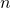subjects. We fit a logistic regression model to binary outcome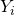with covariates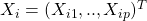. This specifies that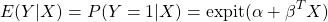where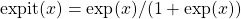. The maximum likelihood estimates of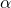and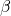are the values solving the so called score equations – the derivative of the log likelihood function with respect to the parameters is set to zero. The likelihood function can be written here as: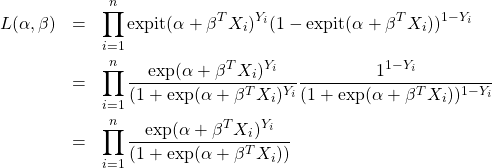so that the log likelihood function is given by: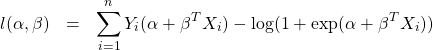If we differentiate with respect towe have: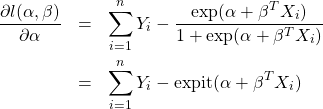Setting this to zero the maximum likelihood estimates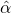and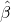then satisfy: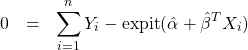If we define the residuals as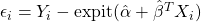, which is the observedminus its predicted value, this means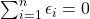, i.e. the sum (and hence also mean) of the residuals is precisely zero.

Re-arranging this equation we can also see that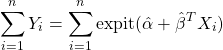or equivalently that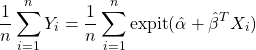This means that the average of the predicted probabilities of Y=1 from the model is exactly equal to the observed proportion of Y=1s in the sample used to fit the model. This property has been referred to as ‘calibration in the large’ in the risk prediction literature.

This site uses Akismet to reduce spam. Learn how your comment data is processed.